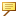Piping > Piping > Setting Up Piping Library > Bend Tables > Conversion Equations
Conversion Equations
The conversion equation tells the system how table data (A) is related to the developed length (L). If no conversion equation is defined, the system assumes that the table data equals the required length (L = A). For example, if the table data represents the bend deduction value, the equation might look like the following:
L = 2*(T + R) - AL must never be negative.
Conversion Equation Variables
You can use the following variables in a conversion equation:
L—Developed straight length of stock.